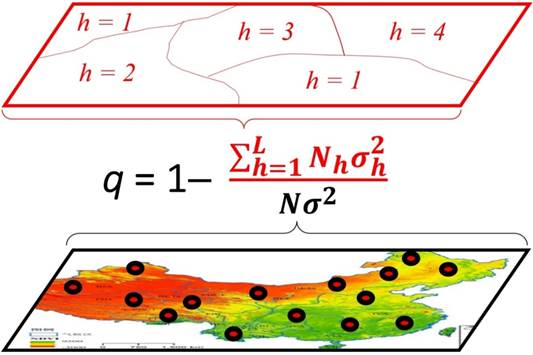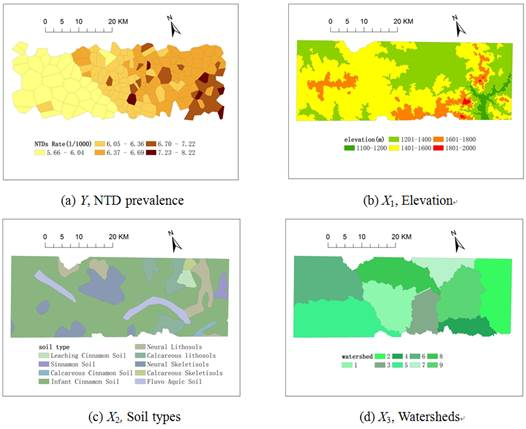## Geographical detectorWays for measuring of spatial stratified heterogeneity (SSH) and attributing of spatial patterns

GeoDetector
195

#### Contributor#### Authorship

Affiliation:
State Key Laboratory of Resources and Environmental Information System
Homepage:
View
Is authorship not correct? Feed back

#### Classification(s)

Geography SubjectHuman GeographyHealth Geography
Geography SubjectGIScience & Remote SensingSpatial Analysis
Geography SubjectGIScience & Remote SensingGeostatistics
Geography SubjectGIScience & Remote SensingGeographic Simulation

#### Detailed Description

Geodetector

Software for measuring of spatial stratified heterogeneity (SSH) and attributing of spatial patterns

# 1.        Introduction

Spatial stratified heterogeneity (SSH) refers to the phenomena that within strata are more similar than between strata, such as well known landuse types and climate zones and those yet to be known, is ubiquitous in universe. As a set of information or patterns, SSH has been a window for humans to recognize the nature and to understand its mechanisms behind since Aristotle time.

Geographical detector is a novel tool to identify SSH and to attribute the spatial patterns (Fig. 1): (1) measure and find SSH of a variable Y; (2) test the association between two variables Y and X, according to the coupling between their spatial distributions, without assumption of linearity; and (3) investigate interaction between two explanatory variables X1 and X2 to a response variable Y, without any specific form such as assumed product in econometrics (Fig. 2). Each of the tasks can be accomplished by the geographical detector q-statistic:Fig. 1. Principle of Geodetector

(The bottom map, the color indicates the values of a population Y; the small circles stand for sample units. The top map, the population Y is stratified into strata {h}; the terms “stratification” and “partition” are equivalent, can be either classification or zonation. Between the two maps is the equation q(Y|{h}), in which the numerator is the summation of the within strata variance and the denominator is the pooled variance.)

where N andstand for the number of units and the variance of Y in a study area, respectively; the population Y is composed of L strata (h = 1, 2, …, L), Nh andstand for the number of units and the variance of Y in stratum h, respectively. The strata of Y (red polygons in Fig.1) are a partition of Y, either by itself h(Y) or by an explanatory variable X which is a categorical h(X). X should be stratified if it is a numerical variable, the number of strata L might be 2-10 or more, according to prior knowledge or a classification algorithm. [(N-L)q]/[(L-1)(1-q)] ~ F(L-1, N-L, g), where g is a non central parameter (Wang et al 2016).

The strata of Y (red polygons in Fig.1) are a partition of Y, either by Y itself or by an explanatory variable XX is a categorical variable or should be stratified if it is a numerical variable. The number of strata L might be 2-10 or more, according to prior knowledge or a classification algorithm. The terms “spatial stratified heterogeneity (SSH)”, “stratification”, “classification” and “partition” are equivalent. The term “spatial” in SSH can be either geospatial or the spatial in mathematics such as time, attributes.

Interpretation of q value (Fig.1).

The value of q is within [0, 1].

(1)  If Y is stratified by Y itself, then q = 0 indicates that Y is not SSH; q = 1 indicates that Y is SSH perfectly; 100q% measures the degree of SSH of Y.

(2)  If Y is stratified by an explanatory variable X, then q = 0 indicates that there is no coupling between Y and Xq = 1 indicates that Y is completely determined by XX explains 100q% of Y. Please notice that the q-statistic measures the association between X and Y, both linearly and nonlinearly.

Geodetector q statistic helps overcome spatial confounding, sample bias and overfitting.

(1)    Confounding arises if applying global models to SSH population, appeared as insignificant or misleading statistical results. The problem can be avoided if SSH is identified (by Geodetector q statistic) then modelling in the strata, separately.

(2)    A sample would be biased to a population if the population is SSH and some of its strata are unsampled. The problem can be solved if SSH is identified (by Geodetector q statistic) then apply stratified sampling or bias remedy models such as Heckman regression and Bshade method.

(3)    Local models aim to overcome heterogeneity but often suffer overfitting and too many parameters to interpret. The problems can be avoided if modelling in strata or stratifying the parameters of a local model then interpreting the parameters in the strata.

Functions of Geodetector:

(1)    The risk detector maps response variable in strata: Y(X);

(2)    The factor detector q-statistic measures the SSH of a variable Y, or the determinant power of an explanatory variable X of Y;

(3)    The ecological detector identifies the difference of the impacts between two explanatory variables X1 ~ X2;

(4)    The interaction detector reveals whether the risk factors X1 and X2 (and more X) have an interactive influence on a response variable Y (Fig.2).Fig. 2. Interaction between explanatory variables X1 and X2 impacting on a response variable Yq(Y|X1X2).

#### How to cite

Jinfeng Wang (2019). Geographical detector, Model Item, OpenGMS, https://geomodeling.njnu.edu.cn/modelItem/95dbf467-4e53-4744-93b3-da7ec680ab62

#### Comment(s)

{{comment.date}}
{{comment.content}}
{{subComm.date}}
{{subComm.content}}

#### Contributor#### Authorship

Affiliation:
State Key Laboratory of Resources and Environmental Information System
Homepage:
View
Is authorship not correct? Feed back

#### QR Code

You can link related `{{typeName}}` from your personal space to this model item, or you can create a new {{typeName.toLowerCase()}}.

Model Content & Service
Remove Remove
{{ props.row.description }}